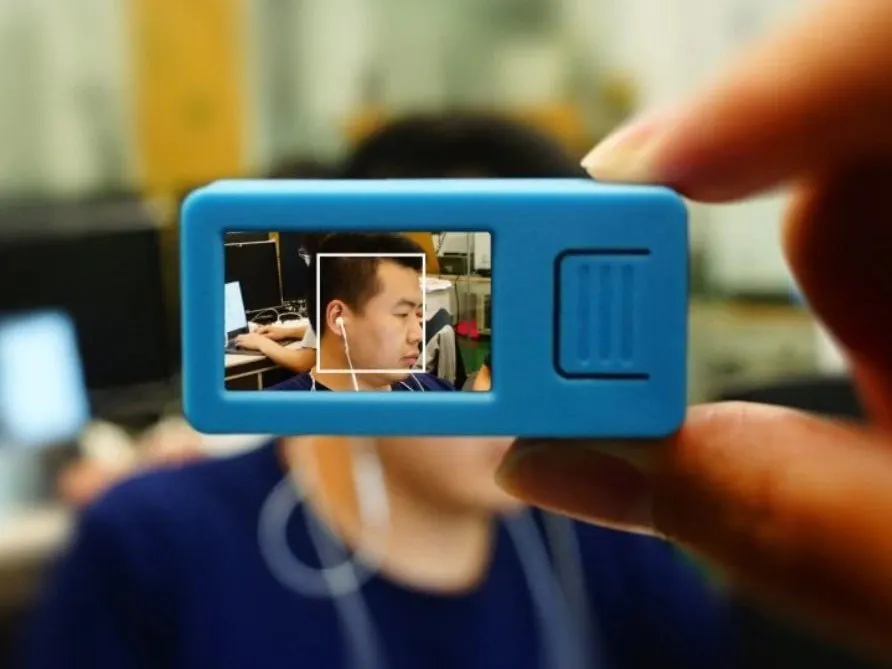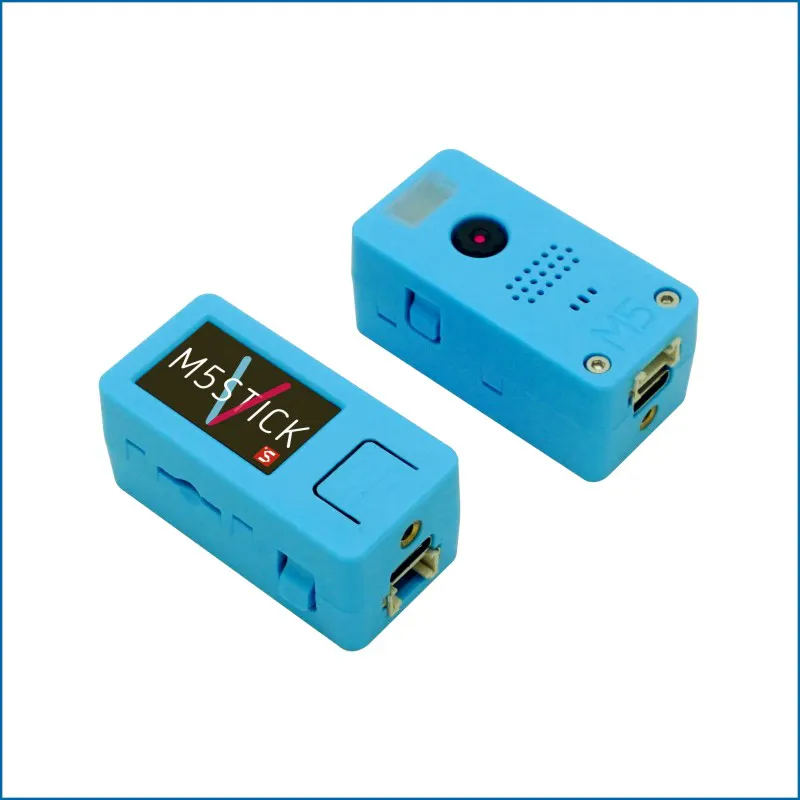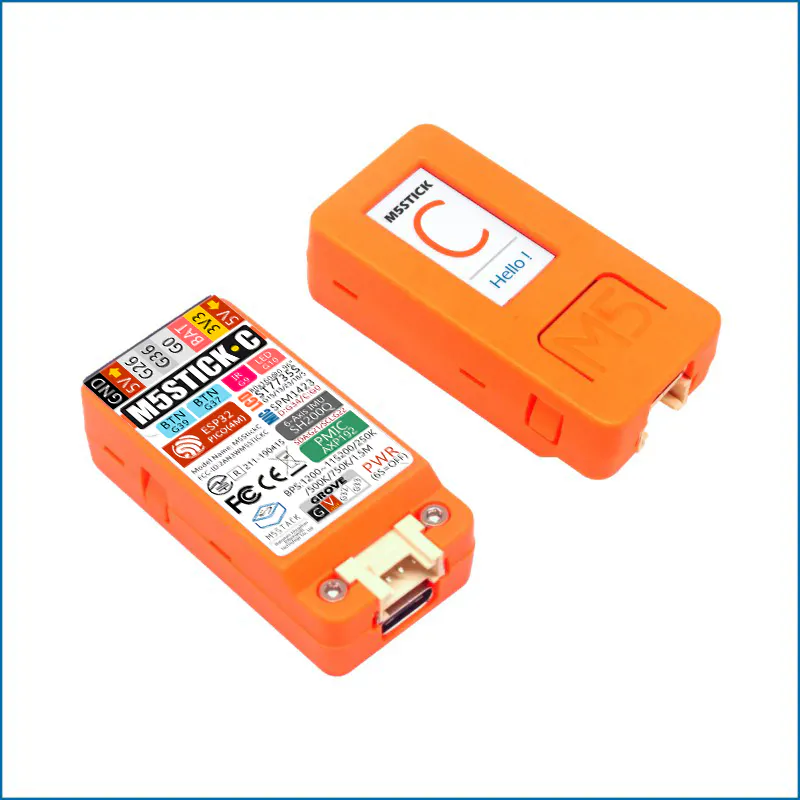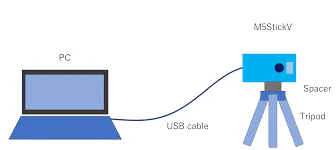# Social Distancing using Face detection + Web Stream

Using M5stickV (UnitV)and its standard Face Detection Model.....Upgrade to enable for Web Stream using M5stickC(ESP32)

IntermediateShowcase (no instructions)1 hour1,993## Things used in this project

### Hardware componentsM5Stack M5StickV K210 AI Camera (Without Wifi)
×1M5Stack M5StickC ESP32-PICO Mini IoT Development Board
×1

### Software apps and online services

 MaixPyIDEArduino IDE

## Schematics

### PC M5stickV Connection## Code

### demo_find_face_social_distancing

MicroPython
Maixpy IDE
```#Reuse the demo find face to create a social distancing....
#Viola variable like a umber of face inbetween
#Viola =3.5 #average distance in between
#Dist distance between two face (center of the face)
#AvgFace smallest diagonal face

import sensor
import image
import lcd
import KPU as kpu
import math
img = image.Image()
lcd.init()
sensor.reset()
sensor.set_pixformat(sensor.RGB565)
sensor.set_framesize(sensor.QVGA)
sensor.run(1)
from array import *

anchor = (1.889, 2.5245, 2.9465, 3.94056, 3.99987, 5.3658, 5.155437, 6.92275, 6.718375, 9.01025)
a = kpu.init_yolo2(task, 0.5, 0.3, 5, anchor)

while(True):
img = sensor.snapshot()
if code:
Xcor=[]
Ycor=[]
Wd=[]
Lh=[]
for i in code:
print(i.index())
print("Cx",i.x()+(i.w()/2))
print("Cy",i.y()+(i.h()/2))

Xcor.insert(i.index(),i.x()+(i.w()/2))
Ycor.insert(i.index(),i.y()+(i.h()/2))
Wd.insert(i.index(),i.w())
Lh.insert(i.index(),i.h())
n=len(Xcor)
print("face detected", n )
a = img.draw_rectangle(i.rect())
for r in range(n-1):
for c in range(0,n-r-1):

Dist = math.sqrt(((Xcor[c] - Xcor[c+1])**2) + (Ycor[c] - Ycor[c+1])**2)

uno =math.sqrt(((Wd[c] )**2) + ((Lh[c])**2))
print("Uno",uno)
dos =math.sqrt(((Wd[c+1] )**2) + ((Lh[c+1])**2))
print("Dos",dos)
AvgFace = uno
if uno >=dos:
AvgFace = dos
print("Average Face Diagonal",AvgFace)
print("Distance",Dist)
Viola =4 #average face distance in between
if ( Viola >= Dist/AvgFace ):
print("ViolatoR", c , c+1)
img.draw_string(int(Xcor[c]), int(Ycor[c]-(Lh[c]*0.25)), "X", scale=3)
img.draw_string(int(Xcor[c+1]), int(Ycor[c+1]-(Lh[c+1]*0.25)), "X", scale=3)
#lcd.draw_string(int(Xcor[c])  , int(Ycor[c])   , "XX", lcd.RED, lcd.BLACK)
#lcd.draw_string(int(Xcor[c+1])  , int(Ycor[c+1])   , "XX", lcd.RED, lcd.BLACK)
del Xcor
del Ycor
del Lh
del Wd
a = lcd.display(img)

```

### M5stickC

Arduino
Act as Access point
Added simplified Streaming Function "mstream" and "mpic" make it ACCESS POINT....<---less lines
```#include <WiFi.h>
#include <WiFiClient.h>
#include <WebServer.h>
#include <ESPmDNS.h>
#include <M5StickC.h>
#include "soc/soc.h" //disable brownout problems
#include "soc/rtc_cntl_reg.h"  //disable brownout problems
//#include "esp_http_server.h"

WebServer server(80);

typedef struct {
uint32_t length;
uint8_t *buf;
} jpeg_data_t;

jpeg_data_t jpeg_data;
static const int RX_BUF_SIZE = 100000;

static const uint8_t packet_begin = { 0xFF, 0xD8, 0xEA };

const char HEADER[] = "HTTP/1.1 200 OK\r\n" \
"Access-Control-Allow-Origin: *\r\n" \
"Content-Type: multipart/x-mixed-replace; boundary=123456789000000000000987654321\r\n";
const char BOUNDARY[] = "\r\n--123456789000000000000987654321\r\n";
const char CTNTTYPE[] = "Content-Type: image/jpeg\r\nContent-Length: ";
const int bdrLen = strlen(BOUNDARY);
const int cntLen = strlen(CTNTTYPE);

void handle_jpg_stream(void)
{
char buf;
int s;

WiFiClient client = server.client();

client.write(BOUNDARY, bdrLen);

while (true)
{//if (!client.connected()) break;

if (Serial2.available()) {
digitalWrite(10, LOW);
uint8_t rx_buffer;
if (rx_size == 10) {
if ((rx_buffer == packet_begin) && (rx_buffer == packet_begin) && (rx_buffer == packet_begin))
{
jpeg_data.length = (uint32_t)(rx_buffer << 16) | (rx_buffer << 8) | rx_buffer;
Serial.println(jpeg_data.length);

client.write(CTNTTYPE, cntLen);
sprintf( buf, "%d\r\n\r\n", jpeg_data.length );
client.write(buf, strlen(buf));
client.write(jpeg_data.buf, jpeg_data.length);
//   client.write((char *)jpeg_data.buf, jpeg_data.length);
client.write(BOUNDARY, bdrLen);

}
}

}

}
}

const char JHEADER[] = "HTTP/1.1 200 OK\r\n" \
"Content-disposition: inline; filename=capture.jpg\r\n" \
"Content-type: image/jpeg\r\n\r\n";

void handle_jpg(void)
{
int state = 1;
WiFiClient client = server.client();

while(state){

//if (!client.connected()) return;
if (Serial2.available()) {
digitalWrite(10, LOW);
uint8_t rx_buffer;
if (rx_size == 10) {
if ((rx_buffer == packet_begin) && (rx_buffer == packet_begin) && (rx_buffer == packet_begin)) {
jpeg_data.length = (uint32_t)(rx_buffer << 16) | (rx_buffer << 8) | rx_buffer;
Serial.println(jpeg_data.length);

client.write(jpeg_data.buf, jpeg_data.length);

//client.write((char *)cam.getfb(), cam.getSize());
}
}
}
}}

void handleNotFound()
{
String message = "Server is running!\n\n";
message += "URI: ";
message += server.uri();
message += "\nMethod: ";
message += (server.method() == HTTP_GET) ? "GET" : "POST";
message += "\nArguments: ";
message += server.args();
message += "\n";
server.send(200, "text / plain", message);
}

void sendPic() {
int state = 1;
WiFiClient client = server.client();

while(state){
if (Serial2.available()) {
digitalWrite(10, LOW);
uint8_t rx_buffer;
if (rx_size == 10) {
if ((rx_buffer == packet_begin) && (rx_buffer == packet_begin) && (rx_buffer == packet_begin)) {
jpeg_data.length = (uint32_t)(rx_buffer << 16) | (rx_buffer << 8) | rx_buffer;
Serial.println(jpeg_data.length);

client.write(jpeg_data.buf, jpeg_data.length);
state = 0;
}
}
}
}
}

void streamPic() {
int state = 1;

while(state){
if (Serial2.available()) {
digitalWrite(10, LOW);
uint8_t rx_buffer;
if (rx_size == 10) {
if ((rx_buffer == packet_begin) && (rx_buffer == packet_begin) && (rx_buffer == packet_begin)) {
jpeg_data.length = (uint32_t)(rx_buffer << 16) | (rx_buffer << 8) | rx_buffer;
Serial.println(jpeg_data.length);

state = 0;
}
}
}
}
WiFiClient client = server.client();

String response = "HTTP/1.1 200 OK\r\n";
response += "Content-Type: multipart/x-mixed-replace; boundary=frame\r\n\r\n";
server.sendContent(response);

while (1)  {
digitalWrite(10, HIGH);

if (Serial2.available()) {
digitalWrite(10, LOW);
uint8_t rx_buffer;
if (rx_size == 10) {
if ((rx_buffer == packet_begin) && (rx_buffer == packet_begin) && (rx_buffer == packet_begin)) {
jpeg_data.length = (uint32_t)(rx_buffer << 16) | (rx_buffer << 8) | rx_buffer;
Serial.println(jpeg_data.length);

}
response = "--frame\r\n";
response += "Content-Type: image/jpeg\r\n\r\n";
server.sendContent(response);

client.write(jpeg_data.buf, jpeg_data.length);
server.sendContent("\r\n");

if (!client.connected())
break;
}else{
break;
}
}

if (!client.connected())
break;
}
}

void setup() {
WRITE_PERI_REG(RTC_CNTL_BROWN_OUT_REG, 0); //disable brownout detector

pinMode(10, OUTPUT);

M5.begin();
M5.Axp.ScreenBreath(0);

Serial.begin(115200);
Serial2.begin(1152000, SERIAL_8N1, 32, 33);

jpeg_data.buf = (uint8_t *) malloc(sizeof(uint8_t) * RX_BUF_SIZE);
/*
WiFi.mode(WIFI_STA);
Serial.println("");

// Wait for connection
while (WiFi.status() != WL_CONNECTED) {
delay(500);
Serial.print(".");
}
Serial.println("");
Serial.print("Connected to ");
Serial.println(ssid);
Serial.println(WiFi.localIP());
*/

//88888888888888888888888888888888
Serial.println();
Serial.println("Configuring access point...");
WiFi.mode(WIFI_AP);                    // Changing ESP32 wifi mode to AccessPoint

// You can remove the Appassword parameter if you want the hotspot to be open.
WiFi.softAP("HELLOO");      //Starting AccessPoint on given credential
IPAddress myIP = WiFi.softAPIP();     //IP Address of our Esp8266 accesspoint(where we can host webpages, and see data)
Serial.println(myIP);                //Default IP is 192.168.4.1

Serial.println("Scan For Wifi in your Mobile or laptop, you will see this network");

if (MDNS.begin("esp32")) {
Serial.println("MDNS responder started");
}

server.on("/", []() {
server.send(200, "text/plain", "this works as well");
});

server.on("/pic", HTTP_GET, sendPic);
server.on("/mpic", HTTP_GET, handle_jpg);
server.on("/stream", HTTP_GET, streamPic);
server.on("/mstream", HTTP_GET, handle_jpg_stream);

server.begin();
Serial.println("HTTP server started");
}

void loop() {
server.handleClient();
}
```

### M5StickV/M5UnitV

Arduino
```#Reuse the demo find face to create a social distancing....
#Viola variable like a umber of face inbetween
#Viola =3.5 #average distance in between
#Dist distance between two face (center of the face)
#AvgFace smallest diagonal face

from machine import UART
from board import board_info
from fpioa_manager import fm
from Maix import GPIO
import sensor, lcd
import image
import KPU as kpu
import math

fm.register(35, fm.fpioa.UART1_TX, force=True)
fm.register(34, fm.fpioa.UART1_RX, force=True)

uart = UART(UART.UART1, 1152000,8,0,0, timeout=1000, read_buf_len=4096)

img = image.Image()
#lcd.init()
sensor.reset()
sensor.set_pixformat(sensor.RGB565)
sensor.set_framesize(sensor.QVGA)
sensor.run(1)
from array import *

anchor = (1.889, 2.5245, 2.9465, 3.94056, 3.99987, 5.3658, 5.155437, 6.92275, 6.718375, 9.01025)
a = kpu.init_yolo2(task, 0.5, 0.3, 5, anchor)

while(True):
img = sensor.snapshot()
if code:
Xcor=[]
Ycor=[]
Wd=[]
Lh=[]
for i in code:
print(i.index())
print("Cx",i.x()+(i.w()/2))
print("Cy",i.y()+(i.h()/2))

Xcor.insert(i.index(),i.x()+(i.w()/2))
Ycor.insert(i.index(),i.y()+(i.h()/2))
Wd.insert(i.index(),i.w())
Lh.insert(i.index(),i.h())
n=len(Xcor)
print("face detected", n )
a = img.draw_rectangle(i.rect())
for r in range(n-1):
for c in range(0,n-r-1):

Dist = math.sqrt(((Xcor[c] - Xcor[c+1])**2) + (Ycor[c] - Ycor[c+1])**2)

uno =math.sqrt(((Wd[c] )**2) + ((Lh[c])**2))
print("Uno",uno)
dos =math.sqrt(((Wd[c+1] )**2) + ((Lh[c+1])**2))
print("Dos",dos)
AvgFace = uno
if uno >=dos:
AvgFace = dos
print("Average Face Diagonal",AvgFace)
print("Distance",Dist)
Viola =4 #average face distance in between
if ( Viola >= Dist/AvgFace ):
print("ViolatoR", c , c+1)
img.draw_string(int(Xcor[c]), int(Ycor[c]-(Lh[c]*0.25)), "X", scale=3)
img.draw_string(int(Xcor[c+1]), int(Ycor[c+1]-(Lh[c+1]*0.25)), "X", scale=3)
#lcd.draw_string(int(Xcor[c])  , int(Ycor[c])   , "XX", lcd.RED, lcd.BLACK)
#lcd.draw_string(int(Xcor[c+1])  , int(Ycor[c+1])   , "XX", lcd.RED, lcd.BLACK)
del Xcor
del Ycor
del Lh
del Wd
img_buf = img.compress(quality=50)
img_size1 = (img.size()& 0xFF0000)>>16
img_size2 = (img.size()& 0x00FF00)>>8
img_size3 = (img.size()& 0x0000FF)>>0
data_packet = bytearray([0xFF,0xD8,0xEA,0x01,img_size1,img_size2,img_size3,0x00,0x00,0x00])

uart.write(data_packet)
uart.write(img_buf)
print("Data Packet",data_packet)

#    a = lcd.display(img)